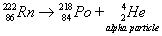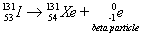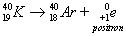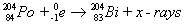##### Biophysics For DummiesCertain naturally occurring radioactive isotopes are unstable: Their nucleus breaks apart, undergoing nuclear decay. Sometimes the product of that nuclear decay is unstable itself and undergoes nuclear decay, too.

For example, when U-238 (one of the radioactive isotopes of uranium) initially decays, it produces Th-234, which decays to Pa-234. The decay continues until, finally, after a total of 14 steps, Pb-206 is produced. Pb-206 is stable, and the decay sequence, or series, stops.

The nucleus has positively charged protons shoved together in an extremely small volume of space. All those protons are repelling each other. The forces that normally hold the nucleus together sometimes can’t do the job, and so the nucleus breaks apart, undergoing nuclear decay.

All elements with 84 or more protons are unstable; they eventually undergo decay. Other isotopes with fewer protons in their nucleus are also radioactive. The radioactivity corresponds to the neutron/proton ratio in the atom:

• If the neutron/proton ratio is too high (there are too many neutrons or too few protons), the isotope is said to be neutron rich and is, therefore, unstable.

• If the neutron/proton ratio is too low (there are too few neutrons or too many protons), the isotope is unstable.

The neutron/proton ratio for a certain element must fall within a certain range for the element to be stable. That’s why some isotopes of an element are stable and others are radioactive.

There are three primary ways that naturally occurring radioactive isotopes decay:

• Alpha particle emission

• Beta particle emission

• Gamma radiation emission

In addition, there are a couple of less common types of radioactive decay:

• Positron emission

• Electron capture

## Alpha emission

An alpha particle is defined as a positively charged particle of a helium nuclei. An alpha particle is composed of two protons and two neutrons, so it can be represented as a Helium-4 atom. As an alpha particle breaks away from the nucleus of a radioactive atom, it has no electrons, so it has a +2 charge. Therefore, it’s a positively charged particle of a helium nuclei.

But electrons are basically free — easy to lose and easy to gain. So normally, an alpha particle is shown with no charge because it very rapidly picks up two electrons and becomes a neutral helium atom instead of an ion.

Large, heavy elements, such as uranium and thorium, tend to undergo alpha emission. This decay mode relieves the nucleus of two units of positive charge (two protons) and four units of mass (two protons + two neutrons). Each time an alpha particle is emitted, four units of mass are lost.

Radon-222 (Rn-222) is another alpha particle emitter, as shown in the following equation:Here, Radon-222 undergoes nuclear decay with the release of an alpha particle. The other remaining isotope must have a mass number of 218 (222 – 4) and an atomic number of 84 (86 – 2), which identifies the element as Polonium (Po).

## Beta emission

A beta particle is essentially an electron that’s emitted from the nucleus. Iodine-131 (I-131), which is used in the detection and treatment of thyroid cancer, is a beta particle emitter:Here, the Iodine-131 gives off a beta particle (an electron), leaving an isotope with a mass number of 131 (131 – 0) and an atomic number of 54 (53 – (-1)). An atomic number of 54 identifies the element as Xenon (Xe).

Notice that the mass number doesn’t change in going from I-131 to Xe-131, but the atomic number increases by one. In the iodine nucleus, a neutron was converted (decayed) into a proton and an electron, and the electron was emitted from the nucleus as a beta particle.

Isotopes with a high neutron/proton ratio often undergo beta emission, because this decay mode allows the number of neutrons to be decreased by one and the number of protons to be increased by one, thus lowering the neutron/proton ratio.

## Gamma emission

Alpha and beta particles have the characteristics of matter: They have definite masses, occupy space, and so on. However, because there is no mass change associated with gamma emission, you could refer to gamma emission as gamma radiation emission.

Gamma radiation is similar to x-rays — high energy, short wavelength radiation. Gamma radiation commonly accompanies both alpha and beta emission, but it’s usually not shown in a balanced nuclear reaction.

Some isotopes, such as Cobalt-60 (Co-60), give off large amounts of gamma radiation. Co-60 is used in the radiation treatment of cancer. The medical personnel focus gamma rays on the tumor, thus destroying it.

## Positron emission

Although positron emission doesn’t occur with naturally occurring radioactive isotopes, it does occur naturally in a few man-made ones. A positron is essentially an electron that has a positive charge instead of a negative charge.

A positron is formed when a proton in the nucleus decays into a neutron and a positively charged electron. The positron is then emitted from the nucleus. This process occurs in a few isotopes, such as Potassium-40 (K-40), as shown in the following equation:The K-40 emits the positron, leaving an element with a mass number of 40 (40 – 0) and an atomic number of 18 (19 – 1). An isotope of argon (Ar), Ar-40, has been formed.

## Electron capture

Electron capture is a rare type of nuclear decay in which an electron from the innermost energy level is captured by the nucleus. This electron combines with a proton to form a neutron. The atomic number decreases by one, but the mass number stays the same.

The following equation shows the electron capture of Polonium-204 (Po-204):The electron combines with a proton in the polonium nucleus, creating an isotope of bismuth (Bi-204). The capture of the 1s electron leaves a vacancy in the 1s orbitals. Electrons drop down to fill the vacancy, releasing energy in the X-ray portion of the electromagnetic spectrum.